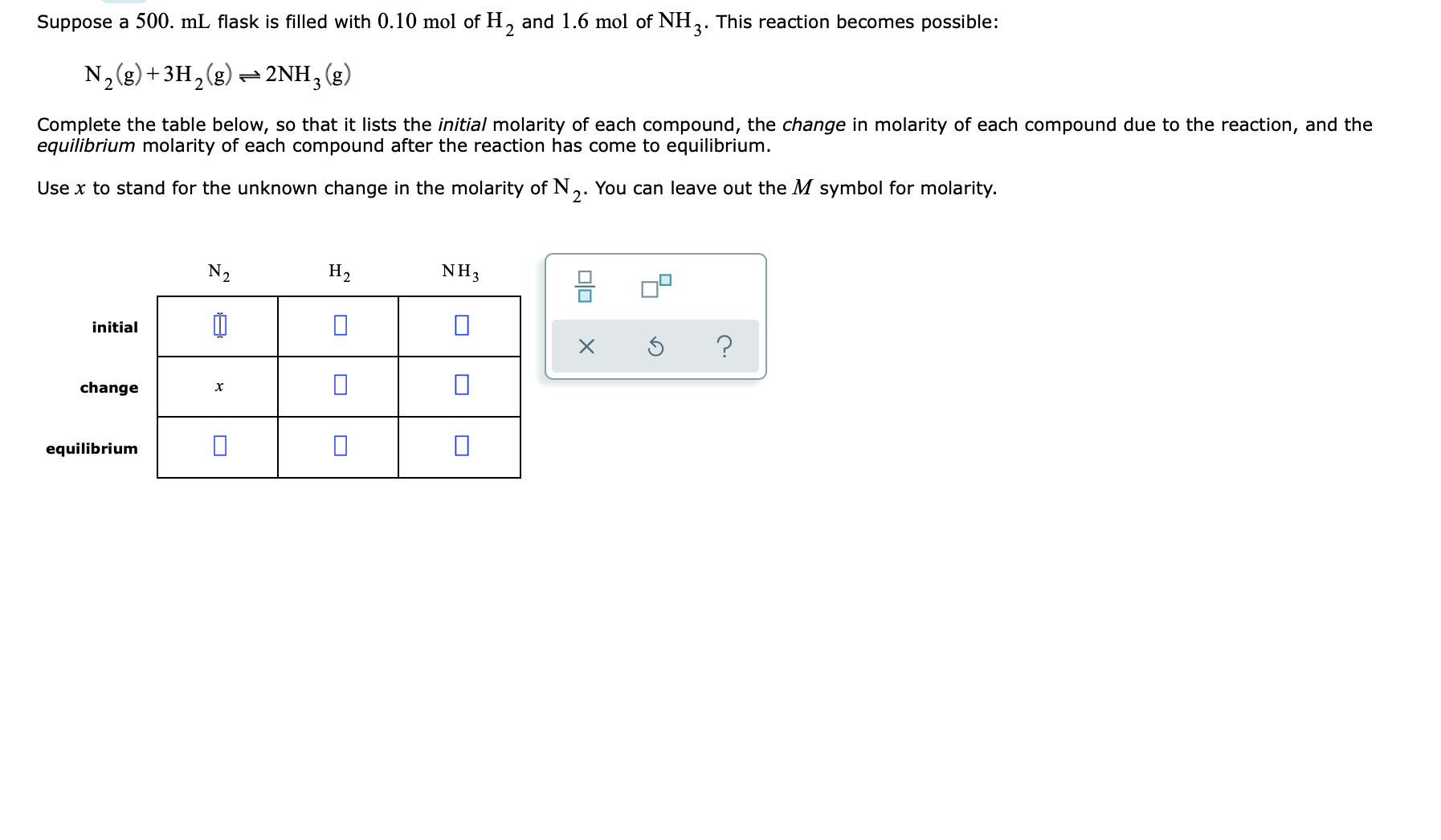# Suppose a 500. mL flask is filled with 0.10 mol of H, and 1.6 mol of NH,. This reaction becomes possible: 3' N,(g) +3H,(g) 2NH,(g) Complete the table below, so that it lists the initial molarity of each compound, the change in molarity of each compound due to the reaction, and the equilibrium molarity of each compound after the reaction has come to equilibrium. Use x to stand for the unknown change in the molarity of N,. You can leave out the M symbol for molarity. N2 H2 NH3 믐 initial change equilibrium

Questionhelp_outlineImage TranscriptioncloseSuppose a 500. mL flask is filled with 0.10 mol of H, and 1.6 mol of NH,. This reaction becomes possible: 3' N,(g) +3H,(g) 2NH,(g) Complete the table below, so that it lists the initial molarity of each compound, the change in molarity of each compound due to the reaction, and the equilibrium molarity of each compound after the reaction has come to equilibrium. Use x to stand for the unknown change in the molarity of N,. You can leave out the M symbol for molarity. N2 H2 NH3 믐 initial change equilibrium fullscreen

### Want to see this answer and more?

Experts are waiting 24/7 to provide step-by-step solutions in as fast as 30 minutes!*

*Response times may vary by subject and question complexity. Median response time is 34 minutes for paid subscribers and may be longer for promotional offers.
Tagged in
Science
Chemistry

### Equilibrium Concepts